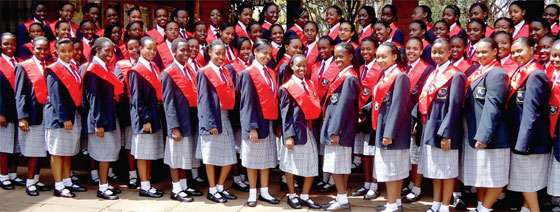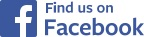## KCSE Exam

At the end of form 4, students take the Kenya Certificate of Secondary Education (KCSE) exam. This is a national exam taken by all form 4 students nationally and at the same time. A students score in this exam determines whether they get to join one of the State Universities and also determines their University courses.

KCSE typically involves taking exams in 8 subjects. Each subject has to exam papers:  paper 1 and paer 2. For the sciences, paper 1 is the theory exam while paper 2 is the practical exam. For the language exam, paper 1 is the grammar and essay exam while paper 2 is the literature exam. Each exam lasts 2 to 4 hours. Each subject has 12 possible points for a possible total of 72 points. The possible scores are as  follows:

 Grade A A- B+ B B- C+ C C- D+ D D- E Points 12 11 10 9 8 7 6 5 4 3 2 1

Scores are described based on the average score in all 8 subjects. Thus a student with an average score of 10 points in the 8 subjects is said to have earned a B+ in the KCSE exam.Talk to us